# Exercise sheet 8 pdfLoading previews...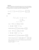eigenvalues and normalised eigenvectors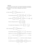diagonalised matrices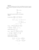system of differential equations, matrices, eigenvalues and eigenvectors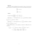system of differential equations, matrices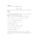system of differential equations, matrices
7 files in this resource

## Exercise sheet 8 pdf

Exercises and solutions in PDFView Item